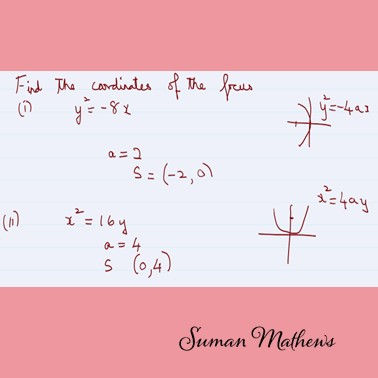top of page
Search

# The Ultimate Guide to Understanding Parabolas on Your Own

## You will first learn how Parabolas originated from Conics and the difference between a Parabola, Ellipse and Hyperbola.Relation between a parabola and conics

## A Parabola is the set of all points in a plane equidistant from a fixed point and a fixed line. The fixed point is called the focus and the fixed line is called the directrix. Problems on these are illustrated below.Simple problem on Parabola

## Next, you will learn the 4 basic types of Parabolas. Left, Right, Top and Bottom, as I tell my students. You will learn how to calculate the equation of the axis, the directrix and the focus for each type. The following image illustrates what you need to remember for the 1st form.Parts of a parabola

## On the other hand, if the vertex is (x + h, y + k), the x axis is shifted h units towards the left and the y axis is shifted k units downwards. You'll need to use this while doing curve sketching in Integration. The following problem illustrates this.problem on shifting of origin